# High School Physics : Understanding Momentum

## Example Questions

### Example Question #1 : Understanding Momentum

A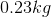baseball approaches a batter at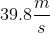. The batter swings and hits a line drive. The ball leaves the bat at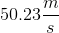. What is the impulse on the ball?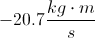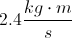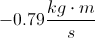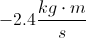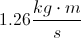Explanation:

Impulse is a change in momentum. Mathematically, we can say that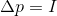.

We can expand this formula to include our terms for mass and velocity.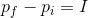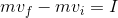The important thing to be aware of in this problem is that we are given the speed of the ball, but not the velocity. Remember, when the baseball leaves the bat it will be going in the OPPOSITE direction. That means its velocity will be in the negative direction.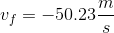Plug in the given information and solve for the impulse.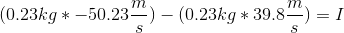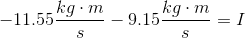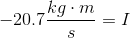It makes since that the impulse would be negative because the change of velocity is an increase in magnitude AND a change in direction.

### Example Question #2 : Understanding Momentum

If an egg is dropped on concrete, it usually breaks. If an egg is dropped on grass, it may not break. What conclusion explains this result?

The density of grass is less than the density of concrete

The egg is in contact with the grass for longer, so it absorbs less force

Grass has less mass than concrete

The coefficient of friction of the grass on the egg is less than the coefficient of the concrete on the egg

Grass is a softer texture

The egg is in contact with the grass for longer, so it absorbs less force

Explanation:

In both cases the egg starts with the same velocity, so it has the same initial momentum. In both cases the egg stops moving at the end of its fall, so it has the same final velocity. The only thing that changes is the time and force of the impact. The force is produced by the deceleration resulting from the time that the egg is in contact with its point of impact.

As the time of contact increases, acceleration decreases and force decreases.

As the time of contact decreases, acceleration increases and force increases.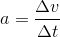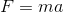The grass will have less force on the egg, allowing for a lesser acceleration, due to a longer period of impact.